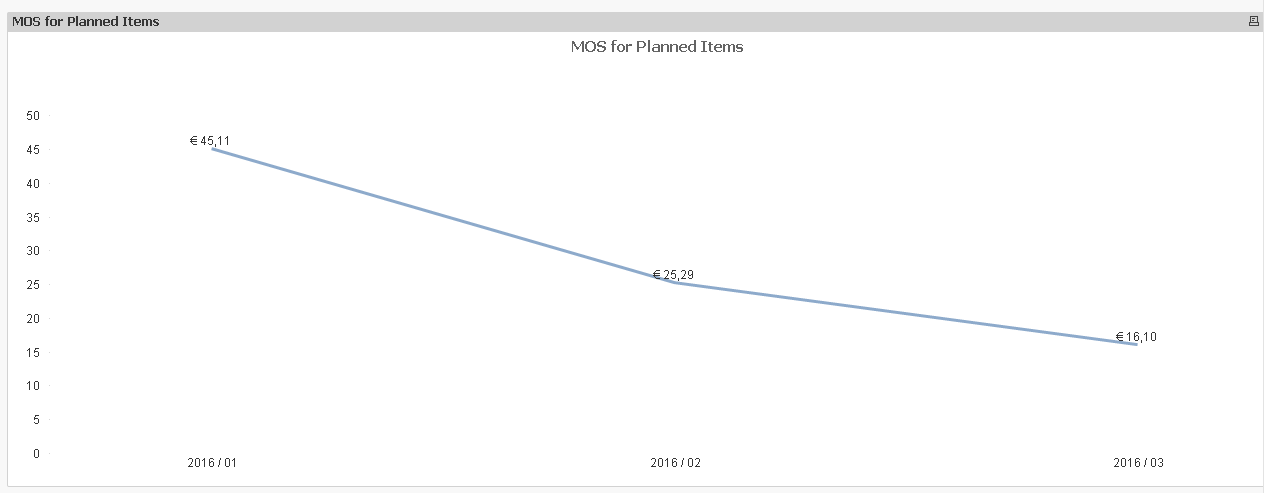# Qlik DataMarket Discussions

Discussion Board for collaboration regarding Qlik DataMarket.Not applicable

## To calculate a value using two different Time attributes

Hi Friends,

I have been struggling to calculate a value using two different Time attributes(Month and Inventory.Month).

The QlikView expression is as follows :-

SUM({\$< Planned ={'Yes'} >}[EUR value])/ rangesum(above(sum([Usage Value]),0,3))

where [Usage Value] is a calculated field in the script.

The field Usage value(measured over Month)  and EUR value(measured over Inventory.Month) are stored in two different tables.

And the time attribute is also different in the two tables.

However, I use dimension  over which I want to calculate the final expression( SUM({\$< Planned ={'Yes'} >}[EUR value])/ rangesum(above(sum([Usage Value]),0,3))) is Inventory.Month.

The QV object does not give any results.

I am not sure how to solve this issue.

Any pointers are highly appreciated.

Thank you !

Tags (2)
1 Solution

Accepted Solutions
HighlightedMVP

## Re: To calculate a value using two different Time attributes

Is this what you want?Expression:

SUM([EUR value])/ rangesum(above(sum([Usage Value]),0,3))

23 Replies
HighlightedMVP

## Re: To calculate a value using two different Time attributes

Are these two tables linked to each other?

Could you post a small sample QVW that better shows your data model?

HighlightedMVP

## Re: To calculate a value using two different Time attributes

May be you need to use Aggr() function where you equate Month to Inventory.Month. Something like this:

Sum({\$< Planned ={'Yes'} >}[EUR value])/ Aggr(If(Month = Innventory.Month, RangeSum(above(sum([Usage Value]),0,3))), AllYourChartDimensions, UniqueIndentifierFromUsageValueTable)

HighlightedNot applicable

## Re: To calculate a value using two different Time attributes

Yes these two tables are linked to each other .

I will try to post the sample qvw for your reference.

Thanks!

HighlightedNot applicable

## Re: To calculate a value using two different Time attributes

This expression does not give any result.

HighlightedMVP

## Re: To calculate a value using two different Time attributes

I guess you are already working on a sample, so there is no use of me going over the above expressionHighlightedNot applicable

## Re: To calculate a value using two different Time attributes

Hi,

HighlightedNot applicable

## Re: To calculate a value using two different Time attributes

I would appreciate if you can also help with the expression because I am interested to understand this logic.

Thank you !

HighlightedMVP

## Re: To calculate a value using two different Time attributes

Is this what you want?Expression:

SUM([EUR value])/ rangesum(above(sum([Usage Value]),0,3))

HighlightedNot applicable

## Re: To calculate a value using two different Time attributes

yes , indeed